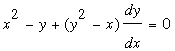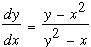SEARCH HOMEMath Central Quandaries & QueriesQuestion from Walter, a student: To whom it may concern, Given x^3 - 3xy + y^3 = 1 , determine y'' by implicitly differentiating twice. I cannot solve this. Would you be kind enough to perform the mathematics and show the steps involved in obtaining the solution? Thank you.Hi Walter,

I differentiated both sides with respect to x, multiplied both sides by 1/3 and was left with.                (1)

Solving for dy/dx gives.                                 (2)

At this point I see two choices, you can differentiate equation 1 or equation 2 to find an expression for the second derivative of y with respect to x. I would differentiate equation 1 as I try to avoid the quotient rule if possible. In either case the expression you obtain will involve the second derivative as well as dy/dx. Substitute for dy/dx using equation 2 and simplify. If you are comparing your answer with one given in the textbook you may need to do some algebra to show they are equivalently as there are different ways to express the result.

HarleyMath Central is supported by the University of Regina and The Pacific Institute for the Mathematical Sciences.